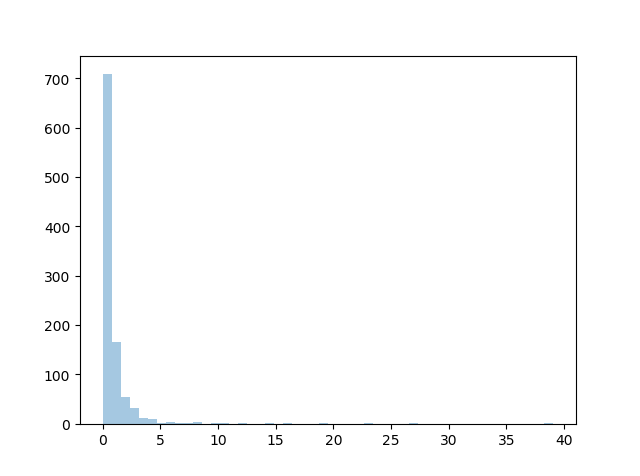THE WORLD'S LARGEST WEB DEVELOPER SITE

# Pareto Distribution

## Pareto Distribution

A distribution following Pareto's law i.e. 80-20 distribution (20% factors cause 80% outcome).

It has two parameter:

`a` - shape parameter.

`size` - The shape of the returned array.

### Example

Draw out a sample for pareto distribution with shape of 2 with size 2x3:

from numpy import random

x = random.pareto(a=2, size=(2, 3))

print(x)
Try it Yourself »

## Visualization of Pareto Distribution

### Example

from numpy import random
import matplotlib.pyplot as plt
import seaborn as sns

sns.distplot(random.pareto(a=2, size=1000), kde=False)

plt.show()

### ResultTry it Yourself »